# Binary Language Explained

Linda Mekhitarian Jackson, Paul Zandbergen
• Author
Linda Mekhitarian Jackson

Linda has a JD from the University of Notre Dame School of Law and a BS in Math and Computer Science from Loyola University Chicago. She has over 15 years experience developing and writing STEM and Humanities curricula, teaching Math, Classics and Literature courses, and conducting ACT/SAT Test Prep classes.

• Instructor
Paul Zandbergen

Paul is a GIS professor at Vancouver Island U, has a PhD from U of British Columbia, and has taught stats and programming for 15 years.

Learn about binary language and its history. Discover how a computer uses binary language and binary codes. Learn about text conversion and binary addition. Updated: 03/11/2022

Show

## Binary Code

Binary code is a system by which numbers, letters and other information are represented using only two symbols, or binary digits. The binary definition to a computer is a 1s and 0s code arranged in ways that the computer can read, understand, and act upon.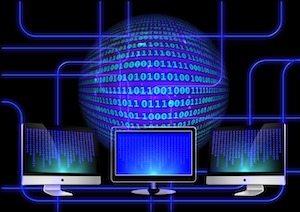As part of its hardware, a computer has a central processing unit, or CPU, consisting of microelectronics, or transistors, that executes instructions. A modern computer's CPU has billions of transistors, or switches, that control the flow of electricity; they can turn an electrical current on or off. When electricity is applied, the switch is on, and the current flows from one electrode through the transistor to the other electrode. If the switch is off, no electricity flows.

The binary number system comes into play because on and off can be represented numerically by 1 and 0. Thus, the off and on positions of an electrical switch inside a computer are physical representations of 1s and 0s. If a switch is on, it is represented by 1, and electricity flows through the transistor. If a switch is off, it is represented by 0, and no electrical current passes through.

When a letter is typed on a keyboard, a signal is sent and converted to binary language which the computer can store and process. This binary code can be explained as machine language which makes sense to the computer. For people to make sense of this binary information, they use a code of 1s and 0s, or binary digits. A binary digit is defined as a bit. A bit can represent either 0 or 1. To represent other values, bits need to be combined. Eight bits comprise a byte, which is the smallest measure used in computer operating systems. Bytes are generally written as two groups of four-bits. The smallest byte is a series of zeroes, 0000 0000, which is the decimal equivalent of zero, and the largest byte is a series of ones, 1111 1111, which is the decimal equivalent of {eq}2^8 {/eq} or 256. It is common to see data referred to in kilobytes, megabytes, gigabytes and terabytes.

Examples of binary codes include alphanumeric codes such as The American Standard Code for Information Interchange (ASCII) and Binary Coded Decimals (BCD), where each decimal digit is represented by a 4-bit binary number.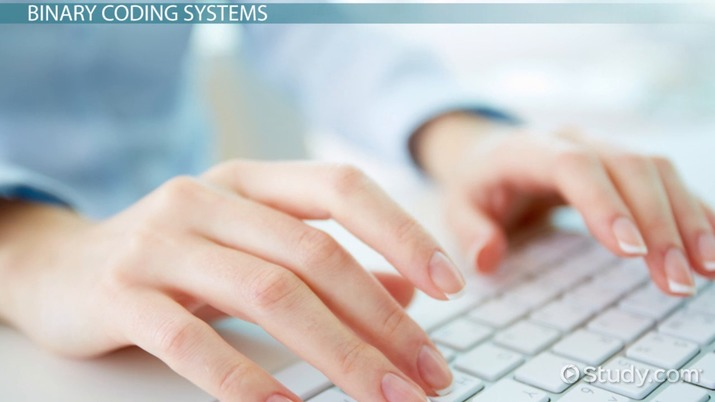An error occurred trying to load this video.

Try refreshing the page, or contact customer support.

Coming up next: Flowchart Symbols in Programming: Definition, Functions & Examples

### You're on a roll. Keep up the good work!

Replay
Your next lesson will play in 10 seconds
• 0:05 Definition
• 1:36 Binary Notation
• 3:36 Binary Coding Systems
• 6:25 Bits and Bytes
• 7:35 Lesson Summary
Save Save

Want to watch this again later?

Timeline
Autoplay
Autoplay
Speed Speed

## History of Binary Codes

When was Binary Invented?

As currently used in computers and devices around the world, binary code was invented when the German polymath, Gottfried Wilhelm Leibnitz (1646-1716), introduced a system of using only the binary digits 0 and 1 to perform arithmetic operations.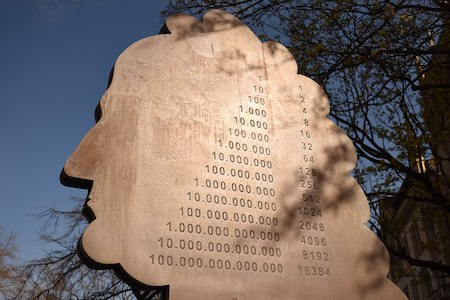Numerical binary schemes existed prior to Leibnitz, but not as comprehensive number systems. Binary language examples include the I-Ching, the ancient Chinese divination text, which used the duality of Yin and Yang for religious and philosophical purposes. The writings of the poet and mathematician, Pindala, illustrate use of a binary system in Indian antiquity. Ancient Egyptians employed a binary system for multiplication and for computation of volume.

Binary codes can also be non-numerical. Francis Bacon (1561-1626) devised a system nearly a century before Leibnitz to represent the letters of the alphabet using five-letter variations of only two letters. Today, this would be called a five-bit binary code and can be thought of more as a secret or encrypted code rather than a system of mathematics.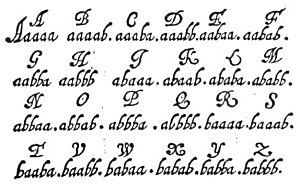Another example of non-numerical binary code is Braille, a writing system of patterns of raised or un-raised dots embossed on paper that the blind can read using their fingers.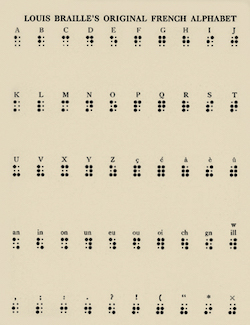## Binary Language

Communicating in binary language requires manipulation of the binary code. Reading binary numbers differs in meaning depending on whether the reader is a computer or a person. Computers read, understand, and act upon binary information. When people refer to reading binary, they generally mean converting the binary, or base 2, numbers into more familiar decimal numbers. When reading binary, it is helpful to understand that the binary system, like the decimal system, is a positional number system, where the value of each digit relies on the position of that digit. Moving from left to right along decimal number, the value of each digit increases by a power of 10, the base. Conversely, moving from right to left results in the opposite, with the value of each digit decreasing by a power of 10. Writing a decimal number in expanded form illustrates this principle: $$2,307 = 2 \times 10^3 +3 \times 10^2 + 0 \times 10^1 +7 \times 10^0$$ Binary numbers work the same way, with the value of each digit changing by power of 2, the base. Accordingly, 11001 in the written in the binary system equals $$1 \times 2^4 + 1 \times 2^3 + 0 \times 2^2 + 0 \times 2^1 + 1 \times 2^0,$$ or 25 in the decimal system (16 + 8 + 1.)

The following chart illustrates how each additional power of 2 creates the subsequent positional column in binary notation.

Decimal 10 Binary 1 1 2 4 8 1000 16 10000

All binary addition is based on four equations: 0 + 0 = 0, 1 + 0 = 1, 0 + 1 = 1, and 1 + 1 = 10. To add 101 + 110 in binary, the operation begins with the ones column and moves left, just like adding decimals, resulting in 1011. This can be double checked using expanded notation.

$$101 = 1 \times 2^2 + 0 \times 2^1 + 1 \times 2^0 = 4 + 0 + 1 = 5$$$$110 = 1 \times 2^2 + 1 \times 2^1 + 0 \times 2^0 = 4 + 2 + 0 = 6$$

$$1011 = 1 \times 2^3 + 0 \times 2^2 + 1 \times 2^1 + 1 \times 2^0 = 8 + 0 + 2 + 1 = 11$$

Since 5 + 6 = 11, the binary addition is correct.

To carry a digit in binary addition, it is helpful to understand that in binary, 1 + 1 + 1 = 10 and that 10 + 1 = 11, resulting in 1 + 1 + 1 = 11. The binary equation 1101 + 1110 = 11011 illustrates this point.

Any kind of data can be represented in binary. In the 1960's, as digital communications began to rival telecommunications, a need arose for standardization so as not to impede interstate commerce. President Johnson decreed The American Standard Code for Information Interchange (ASCII) as the common code to be used for converting characters into binary digits.

To unlock this lesson you must be a Study.com Member.

#### What does 10101 mean in binary?

In binary, 10101 converts to 21 in decimal. This can be written in expanded notation as the following equation: 1 x 2^4 + 0 x 2^3 + 1 x 2^2 + 0 x 2^1 + 1 x 2^0 = 16 + 0 + 4 + 0 + 1 = 21.

#### What is hello in binary?

From the ASCII conversion chart, the letters "HELLO" can be represented by binary numbers as follows:

H - 01001000

E - 01000101

L - 01001100

L - 01001100

O - 01001111

Therefore, HELLO in binary is written as 01001000 01000101 01001100 01001100 01001111.

#### How does binary language work?

All digital data stored and used by computers consists of strings of such binary digits, or bits which can be thought of as machine language. When a letter is typed on a keyboard, a signal is sent to the computer which is converted to strings zeros and ones (binary digits) which the computer can store and process. These zeros and ones can be referred to as machine language which makes sense to the computer.

### Register to view this lesson

Are you a student or a teacher?